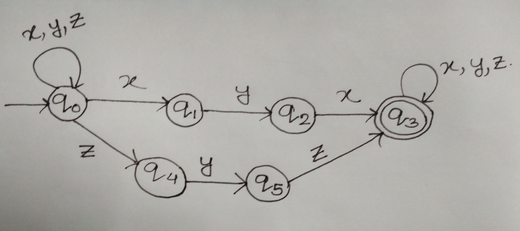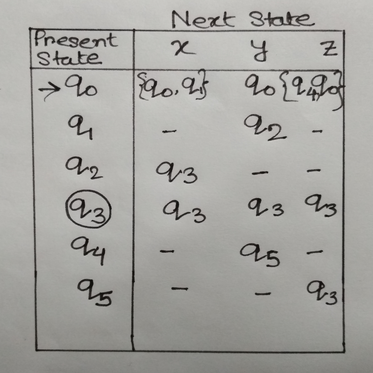# Question & Answer: Write a regular expression for the language……

(1)

Write a regular expression for the language.

Don't use plagiarized sources. Get Your Custom Essay on
Question & Answer: Write a regular expression for the language……
GET AN ESSAY WRITTEN FOR YOU FROM AS LOW AS \$13/PAGE

(2)

Define a finite state machine (FSM) that recognizes words in the language (input alphabet, states, start state, state transition table, and accept states). Include a state digraph for the FSM.

A: For alphabet {x,y,z}, all strings that contain xyx or zyz.

Question (a):

A regular expression is a pattern of text to define regular language.

For this given question the regular expression is:

x*y*z*(xyx + zyz )x*y*z*

Here two operations are used:

• Concatenation to indicate one alphabet followed by another making a sentence.
• Union (+) on a regular expression a + b indicates either a or b.

Another operation * ( also known as kleene closure) is used to denote repetition for zero (0) or more times.

Therefore the minimum string generated from the above regular expression is followed by y followed byor z followed by y followed by z when the there is no repetition for either of x or y or z.

Finite State Automaton:

A finite state automaton is a 5 tuple ( Q, , q0 , F) where Q is the set of states,  is the set of input alphabt,  is a transition function which maps Q X  -> Q ( for Deterministic Finite state Automaton) or Q X  -> 2Q ( for Non-deterministic Finite state Automaton), q0 is the initial state and F is the set of final states.

Question (2):

FSM:Here, NFA is used where Q = { q0 , q1 , q2 , q3, q4, q5}

= {x, y, z}

q0 is the initial state

F=q3

and the transition function is defined in the following table:

State Diagram: